# For the curve y = 4x^3 - 2x^5 find all the points at which tangents passes through origin

For the curve y = 4x3 - 2x5 find all the points at which tangents passes through origin

Let (x1, y1) be point on the curve where tangent is such that it passes through origin. To find slope we need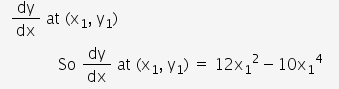So equation of tangent becomes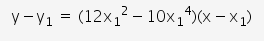This passes through origin
So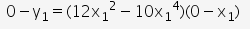On simplification this gives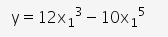But since (x1, y1)lies on curve so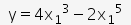Therefore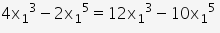So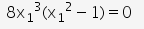Which givesWhen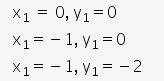So required points are (0, 0), (1, 2) (-1, -2)